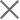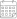Subscribe

### Indexes

April 2023 | Electricity Market Review05 June 2023

In April 2023, Georgian power plants generated 1,249 mln. kWh of electricity. This represents an 11% increase in the total generation compared to the previous year (in April 2022, the total generation was 1,125 mln. kWh). The increase in the generation on a yearly basis comes from an increase of 181%, 38%, and 5% in thermal, wind, and hydropower generation, respectively.

On a monthly basis, the generation increased by approximately 23% (in March 2023, the total generation was 1,019 mln. kWh). The monthly rise in total generation is induced by a 42% increase in hydropower generation and 29% in wind power generation, while thermal power generation decreased by 53%.

The consumption of electricity on the local market was 1,037 mln. kWh (-10% compared to April 2022, and -7% compared to March 2023). In April 2023, power generation exceeded consumption by 212 mln. kWh which was 17% of the total generation and 20% of the total consumption (in April 2022, the difference between the total generation and the consumption resulted in a deficit of 30 mln. kWh, around 3% of the total generation and 3% of the total consumption for the month).